Human Eye and Colourful World - NCERT Questions
Q 1.

What is meant by power of accommodation of the eye?

SOLUTION:

Power of accommodation of eye is the ability of the eye to observe distinctly the objects situated at widely different distances from the eye, on account of change in focal length of eye lens by the action of ciliary muscles holding the lens.

Q 2.

A person with a myopic eye cannot see objects beyond 1.2 m distinctly. What should be the type of the corrective lens used to restore proper vision?

SOLUTION:

The corrective lens should form the image of the far off object at the far point of the myopic person. So, by using the lens formula, we can write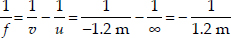or, f = − 1.2 m
So, power of the lens,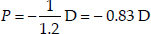Q 3.

What is the far point and near point of the human eye with normal vision?

SOLUTION:

The nearest point up to which an eye can see clearly is called its near point. For a normal eye, the near point is at a distance of 25 cm.
The farthest point up to which an eye can see clearly is called its far point. For a normal eye, the far point is at infinity.

Q 4.

A student has difficulty in reading the blackboard while sitting in the last row. What could be the defect the child is suffering from? How can it be corrected?

SOLUTION:

The student is suffering from myopia. Myopia can be corrected by using glasses made from concave lens of suitable focal length.

Q 5.

The human eye can focus objects at different distances by adjusting the focal length of the eye lens. This is due to
(A) presbyopia
(B) near-sightedness
(C) accommodation
(D) far-sightedness

SOLUTION:

The property of the eye to adjust the focal length of eye lens is called accommodation. Choice (C) is correct.

Q 6.

The human eye forms the image of an object at its
(A) cornea
(B) pupil
(C) iris
(D) retina

SOLUTION:

The human eye forms the image of an object at its retina.

Q 7.

The least distance of distinct vision for a young adult with normal vision is about
(A) 25 m
(B) 2.5 cm
(C) 25 cm
(D) 2.5 m

SOLUTION:

The least distance of distinct vision for a young adult with normal vision is 25 cm.

Q 8.

The change in focal length of an eye lens is caused by the action of
(A) pupil
(B) retina
(C) iris
(D) ciliary muscles

SOLUTION:

The action of ciliary muscles holding the eye lens changes the focal length of eye lens enabling the eye to focus the image of objects at varying distances.

Q 9.

A person needs a lens of power –5.5 dioptres for correcting his distant vision. For correcting his near vision, he needs a lens of power + 1.5 dioptre. What is the focal length of the lens required for correcting (A) distant vision, and (B) near vision?

SOLUTION:

For the near-sighted person, the power of the lens used for distant-viewing is –5.5 D.
So, For distance viewing
=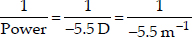= – 0.18 m = –18 cm
Since the near-viewing section of the lens is corrected by + 1.5 D,
∴ Focal length of near vision correction,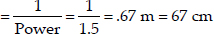Q 10.

The far point of a myopic person is 80 cm in front of the eye. What is the nature and power of the lens required to correct the problem?

SOLUTION:

Here, concave lens is required.
The corrective concave lens should form the image of the far off objects i.e. lying at infinity at the far point of the myopic person. So, using the lens formula,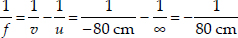So, f = − 80 cm
So, Power of the lens,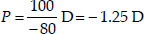Q 11.

Make a diagram to show how hypermetropia is corrected. The near point of a hypermetropic eye is 1 m. What is the power of the lens required to correct this defect? Assume that the near point of the normal eye is 25 cm.

SOLUTION:

The diagram to show the correction of hypermetropia is given here.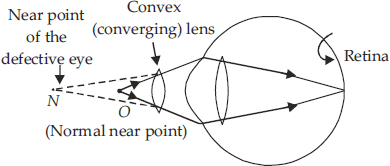Given: Near point distance for a hypermetropic eye, D = 100 cm
Near point distance for a normal eye, D = 25 cm
So, v = – 100 cm, u = – 25 cm
Using the lens formula,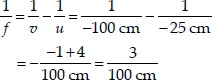So,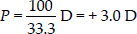Q 12.

Why is a normal eye not able to see clearly the objects placed closer than 25 cm?

SOLUTION:

The least distance of distinct vision for a normal eye is 25 cm. So, a normal eye will not be able to see clearly any object placed closer than 25 cm.

Q 13.

What happens to the image distance in the eye when we increase the distance of an object from the eye?

SOLUTION:

For a normal eye, image distance in the eye is fixed and is equal to the distance of retina from the eye lens. When we increase the distance of the object from the eye, focal length of eye lens is changed on account of accommodating power of the eye so as to keep image distance constant.

Q 14.

Why do stars twinkle?

SOLUTION:

Due to wind and convection currents, density of the atmospheric layers keeps on changing. As a result, the position of a star keeps fluctuating from its mean position. This fluctuating image of the stars makes them appear twinkling to the observer.

Q 15.

Explain why the planets do not twinkle.

SOLUTION:

Planets do not emit light. However, they become visible due to reflection of light falling on them. The planets are much closer to the earth and thus can be considered as the extended source of light.
The fluctuations in the light coming from various points of the planet due to atmospheric refraction get averaged out. As a result, no twinkling of planets is seen.

Q 16.

Why does the sun appear reddish early in the morning?

SOLUTION:

At sunrise and sunset, the sun is closer to the horizon. The sunlight near the horizon passes through denser layers of the air and covers larger distance before reaching our eyes. Most of the blue light gets scattered. The light that reaches our eyes is of longer wavelengths, mainly orange and red. That is why the sun appears red at the sunrise and at the sunset.

Q 17.

Why does the sky appear dark instead of blue to an astronaut?

SOLUTION:

This is because at such huge heights of the astronaut, there is nothing to scatter the sunlight. Therefore the sky appears dark.